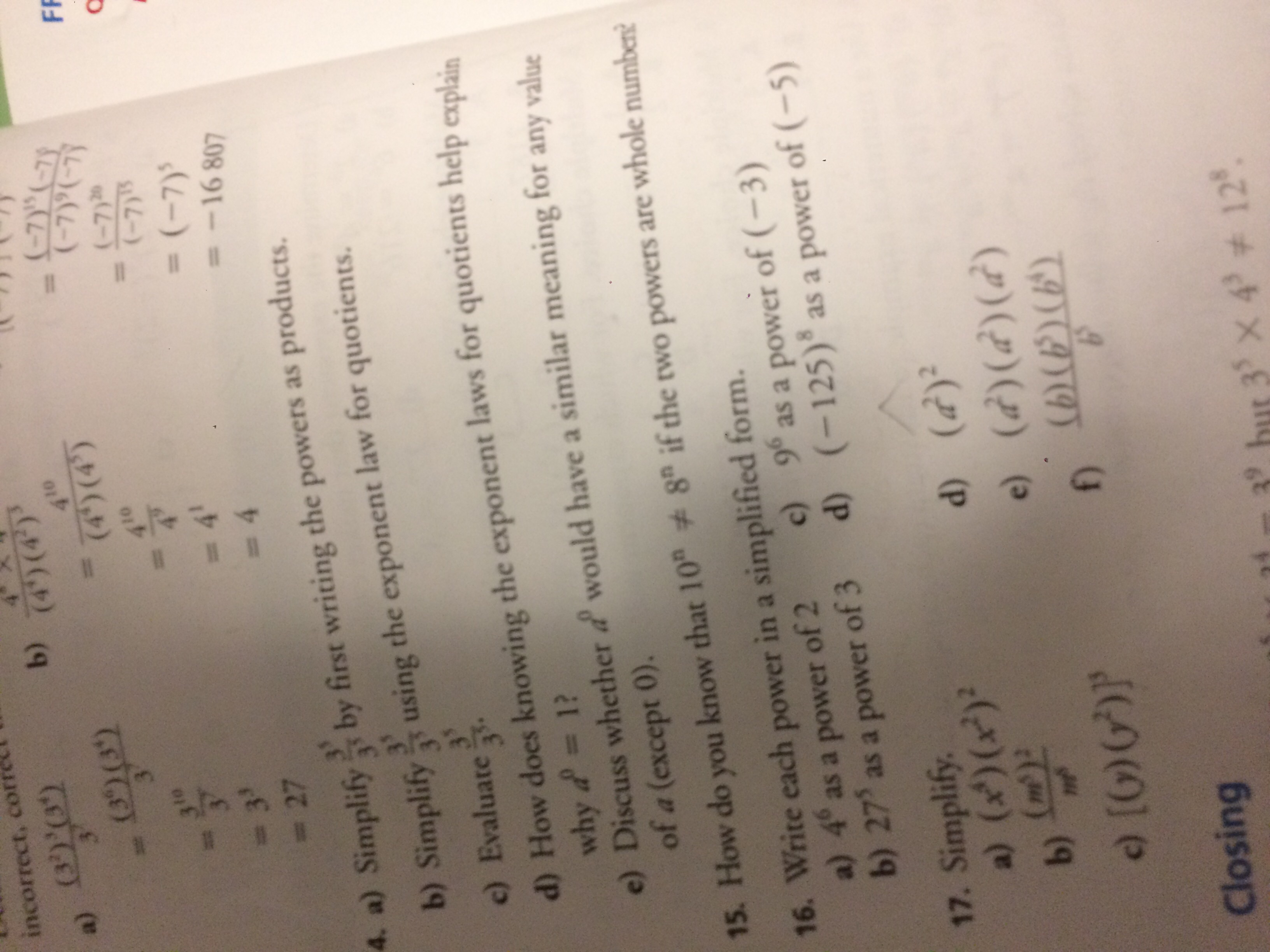# # ! II# incorrect, correl a) (3)'09 3 b) (ব)() 410 (4')(4) (7y (-7( (3)(3) FR 10 (-7)2 (-7)5 3 3 - 27 4 = (-7) = 4 -16 807 4. a) Simplify by first writing the powers as products. using the exponent law for quotients. b) Simplify 35 3 c) Evaluate d) How does knowing the exponent laws for quotients help explain why d 1? e) Discuss whether d would have a similar meaning for any value of a (except 0). t 15. How do you know that 10 8 if the two powers are whole numben 16. Write each power in a simplified form. a) 4 as a power of 2 b) 27' as a power of 3 d) (-125) as a power of (-5) c) 9 as a power of (-3) 17. Simplify. a) (x)(x) (m) d) (d) e) (d)(d)(d) (b(6)() b) [0)6)P f) Closing X 4 12

Question

I just need help on how to solve number 16.help_outlineImage Transcriptionclose# ! II# incorrect, correl a) (3)'09 3 b) (ব)() 410 (4')(4) (7y (-7( (3)(3) FR 10 (-7)2 (-7)5 3 3 - 27 4 = (-7) = 4 -16 807 4. a) Simplify by first writing the powers as products. using the exponent law for quotients. b) Simplify 35 3 c) Evaluate d) How does knowing the exponent laws for quotients help explain why d 1? e) Discuss whether d would have a similar meaning for any value of a (except 0). t 15. How do you know that 10 8 if the two powers are whole numben 16. Write each power in a simplified form. a) 4 as a power of 2 b) 27' as a power of 3 d) (-125) as a power of (-5) c) 9 as a power of (-3) 17. Simplify. a) (x)(x) (m) d) (d) e) (d)(d)(d) (b(6)() b) [0)6)P f) Closing X 4 12 fullscreen

### Want to see this answer and more?

Experts are waiting 24/7 to provide step-by-step solutions in as fast as 30 minutes!*